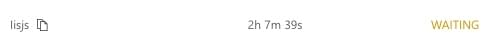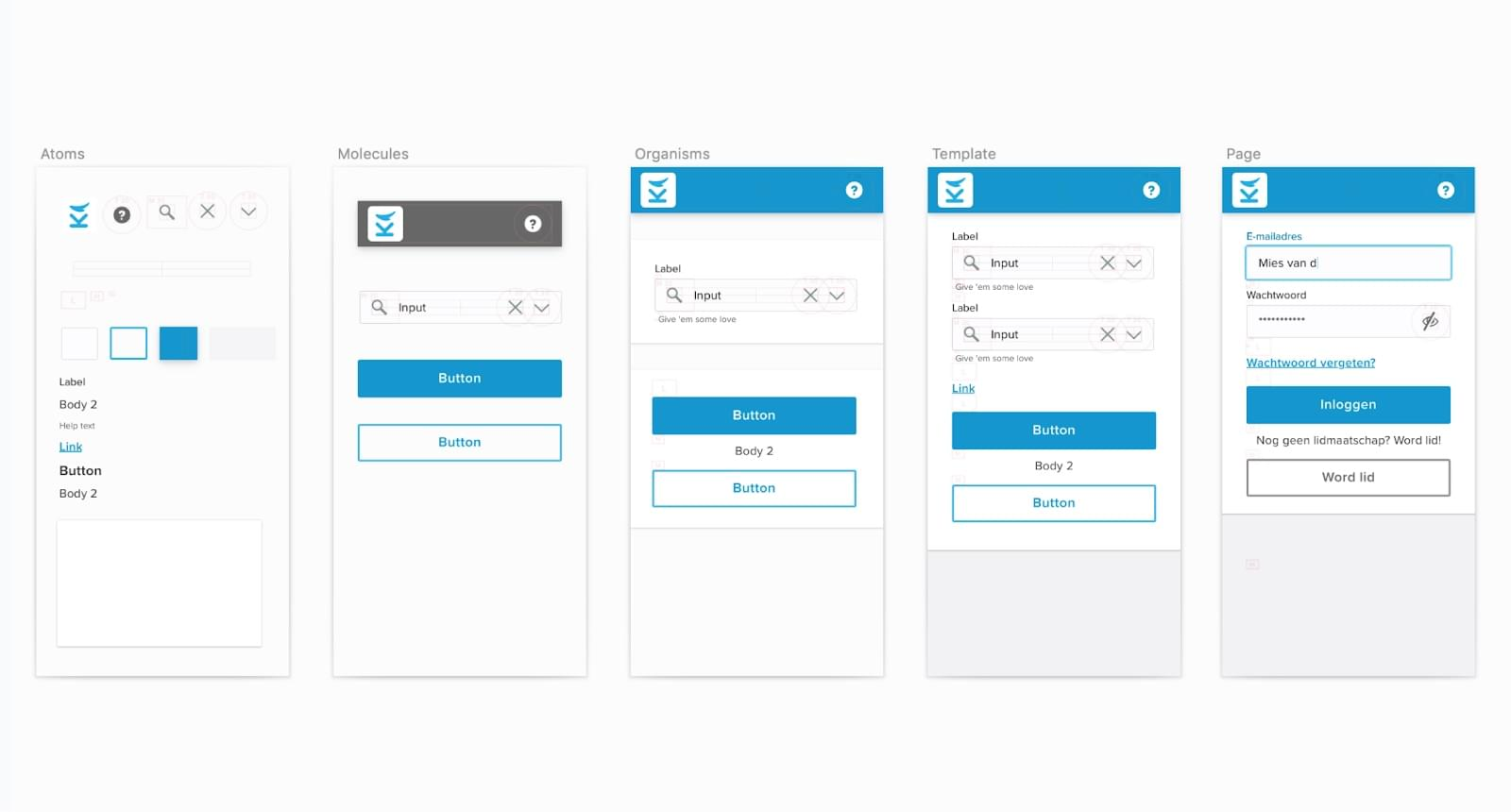0%

## 问题定义

1. 触发词检测：触发词（Trigger）是事件抽取中的重要信息，一般是出现在事件提及中，最能明确表达发生事件的词，一般是动词或名词。例如，"20年前的春天，他出生了"一句中，"出生"为该事件提及文本中的事件触发词。
2. 事件类型检测：即通过分类等方式得到事件的类型，由于触发词在事件中的关键性，因此也可以被视作触发词类型检测。事件的类型取决于事件模式的设计，或事理图谱schema的设计。例如，某事件模式中将"咬伤"、"砍死"等触发词的事件定义为"伤害"类型的事件。
3. 事件论元抽取：事件论元（Event Argument）指的是事件中的参与者，包含实体、时间、数值、文本等数据组成。例如，"张三在2022年成功晋升"中的张三、2022年均为"晋升"事件的事件论元。
4. 论元角色识别：根据事件模式或事理图谱的定义，将抽取的事件论元按照其在事件中扮演的具体角色进行分类。例如，在某"公安事理图谱"中的"张三"、"李四"等人在其对应的犯罪记录中均为"加害者"这一事件角色。

1. 事件分割：也有称故事分割，给定一段文本（如新闻、论坛发言等），检测出不同事件的边界。例如，在央视"今日新闻简讯"中包含了当日多条要闻，有的新闻条目使用了多段文本描述，有的文本段落中一次包含了多条新闻，将它们分离成独立的事件文本片段即为本任务的目标。
2. 事件发现：在新闻、论坛发言等文本中，检测出新的事件（New Event Detection）。常用于舆情系统等应用。
3. 事件追踪：在新闻、论坛发言等文本中，检测同属于之前的已有事件的文本片段，通过此方式追踪事件（Event Tracking）的发展情况。常用于舆情系统等应用。

Enhancing Chinese Pre-trained Language Model via Heterogeneous Linguistics Graph 一文是我和组内同学合作的工作，录用于 ACL 2022 主会。代码已在 GitHub开源，ppt可在这里查看。

## 问题背景

MWA是利用一种非标准形式的分段式attention方法，将中文的分词切割信息应用到字符表示产生的attention权重上，对同一个词中的不同字进行mix-pooling聚合，从而让字的attention权重强行在词的级别上进行对齐。这样的设计有效地融入了分词的分段式的结构信息，但也带来了一些新的问题：由于需要逐词、逐样本地计算attention的聚合，会导致attention模型中原本可以向量化、并行化的标准矩阵运算变成需要各自运算、无法并行的高负载运算，并且这样的算子无法利用cudnn原语的加速，也无法享受当今非常重要的深度学习计算加速硬件（如GPU、TPU等）带来的速度提升。

## 方法原理

HLG的构图方法在实质上就满足了前面提到的去噪的动机。以上图中“西山”节点，和“西”、“山”节点为例，前者（西山）有两个分词器支撑这个分词结果，而后者（西、山）只有一个分词器支撑，前者产生的节点在图中的度数会比后者更高。

## 总结

•  Enhancing Pre-trained Chinese Character Representation with Word-aligned Attention, ACL 2020.
•  Ernie: Enhanced representation through knowledge integration, ACL 2019.
•  Glyce: Glyph-vectors for chinese character representations, NIPS 2019.
•  Deep Residual Learning for Image Recognition, CVPR 2016.

## 引言

### 融合子图的构建

（1）从知识库构建问题相关子图：首先通过实体链指从问题$q$中获取一系列种子实体$S_q$，然后以这些种子实体为起点，执行Personal PageRank（PPR）算法，根据PPR得到的权重找出与种子实体相连的Top-E候选实体$v_{1}, \ldots, v_{E}$，将种子实体、候选实体和他们间的连边合起来，构成子图$\mathcal{G}_q = \left(\mathcal{V}_{q}, \mathcal{E}_{q}, \mathcal{R}\right)$，其中$\mathcal{V}$为节点，$\mathcal{E}$为连边，$\mathcal{R}$为边的类型（即关系类型）。

（2）文本信息节点：首先通过DrQA的带权词袋模型对文本库进行句子级别的检索，得到Top5与问题相关的文档，然后根据问题$q$与文档中的句子进行检索排序，找出最相关的Top-D个候选句子$d_{1}, \ldots d_{D}$。将这Top-D个句子依次加入问题相关子图$\mathcal{G}_q$中： $\mathcal{V}_{q}=\left\{v_{1}, \ldots, v_{E}\right\} \cup\left\{d_{1}, \ldots, d_{D}\right\}$ 对于节点的连边，以如下的形式进行连接： $\mathcal{E}_{q}=\left\{(s, o, r) \in \mathcal{E}: s, o \in \mathcal{V}_{q}, r \in \mathcal{R}\right\} \cup\left\{\left(v, d_{p}, r_{L}\right):\left(v, d_{p}\right) \in \mathcal{L}_{d}, d \in \mathcal{V}_{q}\right\}$

### 图表示学习与节点分类

（1）初始化节点表示：对于知识库中的实体节点，赋予定长表示向量：$h_{v}^{(0)}=x_{v} \in \mathbb{R}^{n}$，其中$x_v$可以是随机向量也可以是通过TransE等方式预训练得到表示向量。对于文本的文档节点，如果文档是由单词$w_{1}, \ldots, w_{|d|}$构成的，则使用LSTM对词嵌入进行编码$H_{d}^{(0)}=\operatorname{LSTM}\left(w_{1}, w_{2}, \ldots\right)$

（2）异构融合子图的更新：由于前一步得到的图是异构的，需要根据问题$q$分别对实体节点与文档节点进行更新，如上图所示。对于实体节点$v$，每一层GRAFT-Net执行如下更新： $h_{v}^{(l)}=\mathrm{FFN}\left(\left[\begin{array}{c} h_{v}^{(l-1)} \\ h_{q}^{(l-1)} \\ \sum_{r} \sum_{v^{\prime} \in N_{r}(v)} \alpha_{r}^{v^{\prime}} \psi_{r}\left(h_{v^{\prime}}^{(l-1)}\right) \\ \sum_{(d, p) \in M(v)} H_{d, p}^{(l-1)} \end{array}\right]\right)$ 其中$h_{v}^{(l-1)}$是上一层该节点的表示，$h_{q}^{(l-1)}$是上一层的问题表示，$\sum_{r} \sum_{v^{\prime} \in N_{r}(v)} \alpha_{r}^{v^{\prime}} \psi_{r}\left(h_{v^{\prime}}^{(l-1)}\right)$中的$N_{r}(v)$是节点$v$的邻居实体节点，$\psi_{r}\left(h_{v^{\prime}}^{(l-1)}\right)$是根据PageRank的权重控制连边上的权重传递（propagation），$\alpha_{r}^{v^{\prime}}$是在关系$r$上执行注意力机制加权，以权衡问题节点对实体节点的影响；$\sum_{(d, p) \in M(v)} H_{d, p}^{(l-1)}$中的$M(v)$是与节点$v$相邻的文档节点，$H_{d, p}^{(l-1)}$是实体在文本中的表示。 而文档节点的表示，是由一系列单词的表示再通过LSTM进行编码得到的，因此文档节点表示的更新本质上是文档中单词表示的更新。假如文档$d$中位置$p$上的单词与实体节点相连，将这些实体节点记为$L(d, p)$，该单词的表示更新如下所示： $\tilde{H}_{d, p}^{(l)}=\operatorname{FFN}\left(H_{d, p}^{(l-1)}, \sum_{v \in L(d, p)} h_{v}^{(l-1)}\right)$ 实质上就是对邻接实体节点进行了聚合。最后，文档节点也会更新：$H_{d}^{(l)}=\operatorname{LSTM}\left(\tilde{H}_{d}^{(l)}\right)$

《Question Answering over Freebase with Multi-Column Convolutional Neural Networks》发表于 ACL 2015，这篇论文是《Question Answering with Subgraph Embeddings》的后续工作。

（1）答案路径：对于从问题中的实体节点到答案节点的路径的表示，此论文采用了和《基于子图嵌入的知识库问答》中路径表示一样的方法： $\mathbf{g}_{1}(a)=\frac{1}{\left\|\mathbf{u}_{p}(a)\right\|_{1}} \mathbf{W}_{p} \mathbf{u}_{p}(a)$

（2）答案上下文（Context）：该文将与答案相邻一跳的实体和关系称为答案的上下文，使用同样的方法进行嵌入表示： $\mathbf{g}_{2}(a)=\frac{1}{\left\|\mathbf{u}_{c}(a)\right\|_{1}} \mathbf{W}_{c} \mathbf{u}_{c}(a)$

（3）答案类型：作者认为，类型信息对于知识库问答十分重要，可以根据问题直接限定到答案的类型。例如当问题中有“Where”的时候，答案也应有很大的可能是与“location”相关的类型。因此，作者对答案的类型进行了与前文类似的表示： $\mathbf{g}_{3}(a)=\frac{1}{\left\|\mathbf{u}_{t}(a)\right\|_{1}} \mathbf{W}_{t} \mathbf{u}_{t}(a)$

《Question Answering with Subgraph Embeddings》发表于 2014 年的 EMNLP，提出了一种基于对问题嵌入与候选子图嵌入进行打分的排序学习的方法，是基于信息检索的知识库问答中比较有代表性的工作。

（1）实体嵌入：与问题嵌入的方式相同，直接通过实体 one-hot 在共享的词嵌入参数矩阵中获取对应的嵌入： $g(a)=\mathbf{W} \psi(a),$ 其中$\psi(a)$为与$\phi(q)$类似的稀疏统计向量，会将答案以$N_S$维的稀疏向量表示，$g(a)$为获得的实体嵌入（即答案嵌入）。如果一个问题存在多个答案，则直接对多个答案的嵌入表示求平均。

（2）路径嵌入：该工作会考虑最多两跳的答案路径。例如对一个两跳的答案路径：(barack obama, people.person.place of birth, location. location.containedby, hawaii)中的头实体、尾实体和路径上的所有谓词都使用$\psi(a)$进行嵌入。

（3）子图嵌入：对一个答案，在一跳或两跳的范围内构建子图，对这个子图中包含的实体和关系同样使用$\psi(a)$进行嵌入。但为了区分答案路径与子图中实体和关系，作者对$N_S$设定了两倍的大小，子图和路径的嵌入分别使用不同的部分。